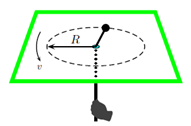Problem: A small puck moves in a circle on a frictionless air table. The circular motion is enforced by a person holding a string tied to the puck and going through a tiny hole in the middle of the table. Initially, the puck moves in a circle of radius R at speed v. But later the string is pulled down through the hole forcing the puck to move in a smaller circle of radius 1/2 R. How much work did the person do while moving the puck from R to 1/2 R? 1. W =3/4 mv2 2. W =3/2 mv2 3. W = mv2 4. W =5/2 mv2 5. W =2/3 mv2 6. W =1/3 mv2 7. W =4/3 mv2 8. W = 2mv2 9. W =1/4 mv2 10. W =1/2 mv2

93% (90 ratings)
Problem Details

A small puck moves in a circle on a frictionless air table. The circular motion is enforced by a person holding a string tied to the puck and going through a tiny hole in the middle of the table. Initially, the puck moves in a circle of radius R at speed v. But later the string is pulled down through the hole forcing the puck to move in a smaller circle of radius 1/2 R. How much work did the person do while moving the puck from R to 1/2 R?

1. W =3/4 mv2

2. W =3/2 mv2

3. W = mv2

4. W =5/2 mv2

5. W =2/3 mv2

6. W =1/3 mv2

7. W =4/3 mv2

8. W = 2mv2

9. W =1/4 mv2

10. W =1/2 mv2